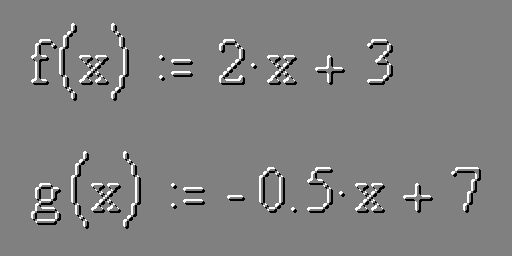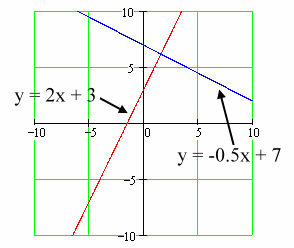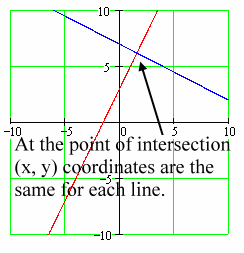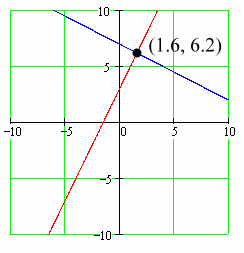# Intersection of Two LinesHere we will cover a method for finding the point of intersection for two linear functions. That is, we will find the (x, y) coordinate pair for the point were two lines cross.

Our example will use these two functions:

f(x) = 2x + 3

g(x) = -0.5x + 7

We will call the first one Line 1, and the second Line 2. Since we will be graphing these functions on the x, y coordinate axes, we can express the lines this way:

y = 2x + 3

y = -0.5x + 7

Note that these two lines are in slope-intercept form.

These two lines look this way:Now, where the two lines cross is called their point of intersection. Certainly this point has (x, y) coordinates. It is the same point for Line 1 and for Line 2. So, at the point of intersection the (x, y) coordinates for Line 1 equal the (x, y) coordinates for Line 2.Since at the point of intersection the two y-coordinates are equal, (we'll get to the x-coordinates in a moment), let's set the y-coordinate from Line 1 equal to the y-coordinate from Line 2.

The y-coordinate for Line 1 is calculated this way:

y = 2x + 3

The y-coordinate for Line 2 is calculated this way:

y = -0.5x + 7

Setting the two y-coordinates equal looks like this:

2x + 3 = -0.5x + 7

Now, we do some algebra to find the x-coordinate at the point of intersection:

 2x + 3 = -0.5x + 7 We start here. 2.5x + 3 = 7 Add 0.5x to each side. 2.5x = 4 Subtract 3 from each side. x = 4/2.5 Divide each side by 2.5. x = 1.6 Divide 4 by 2.5.

So, we have the x-coordinate for the point of intersection. It's x = 1.6. Now, let's find the y-coordinate. The y-coordinate can be found by placing the x-coordinate, 1.6, into either of the equations for the lines and solving for y. We will first use the equation for Line 1:

y = 2x + 3

y =2(1.6) + 3

y = 3.2 + 3

y = 6.2

Therefore, the y-coordinate is 6.2. To make sure our calculations are correct, and also to demonstrate a point, we should get the same y-coordinate if we use the equation for Line 2. Let's try that:

y = -0.5x + 7

y = -0.5(1.6) + 7

y = -0.8 + 7

y = 6.2

Well, looks like everything has worked out. The point of intersection for these two lines is (1.6, 6.2). If you look back at the graph, this certainly makes sense:Here's the summary of our methods:

1. Get the two equations for the lines into slope-intercept form. That is, have them in this form: y = mx + b.
2. Set the two equations for y equal to each other.
3. Solve for x. This will be the x-coordinate for the point of intersection.
4. Use this x-coordinate and plug it into either of the original equations for the lines and solve for y. This will be the y-coordinate of the point of intersection.
5. As a check for your work plug the x-coordinate into the other equation and you should get the same y-coordinate.
6. You now have the x-coordinate and y-coordinate for the point of intersection.

Actually, there is nothing special about the functions being linear functions. This method could be used to find the point or points of intersection between many other types of functions. One would express the functions in 'y =' form, set the right side of these forms equal to each other, solve for x, (or x's), and use this x, (or x's), to find the corresponding y, (or y's).

Here's a calculator to help you check your work. Make up two linear functions in slope-intercept form. Calculate the point of intersection using the above methods. Then enter the slope and y-intercept for each line into the calculator and click the button to check your work.

 Line 1 Slope: Y-intercept: Line 2Slope: Y-intercept:

Custom Search